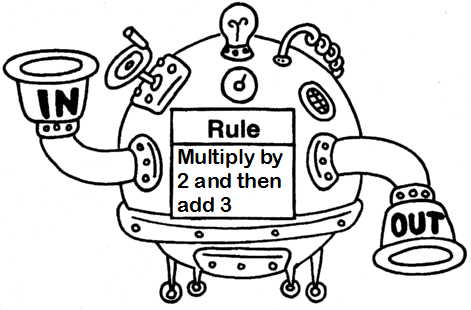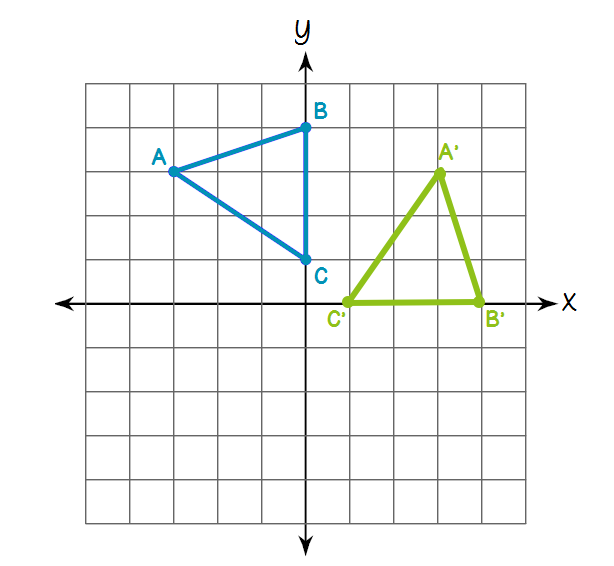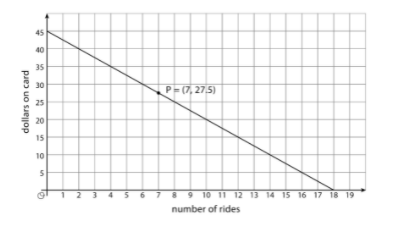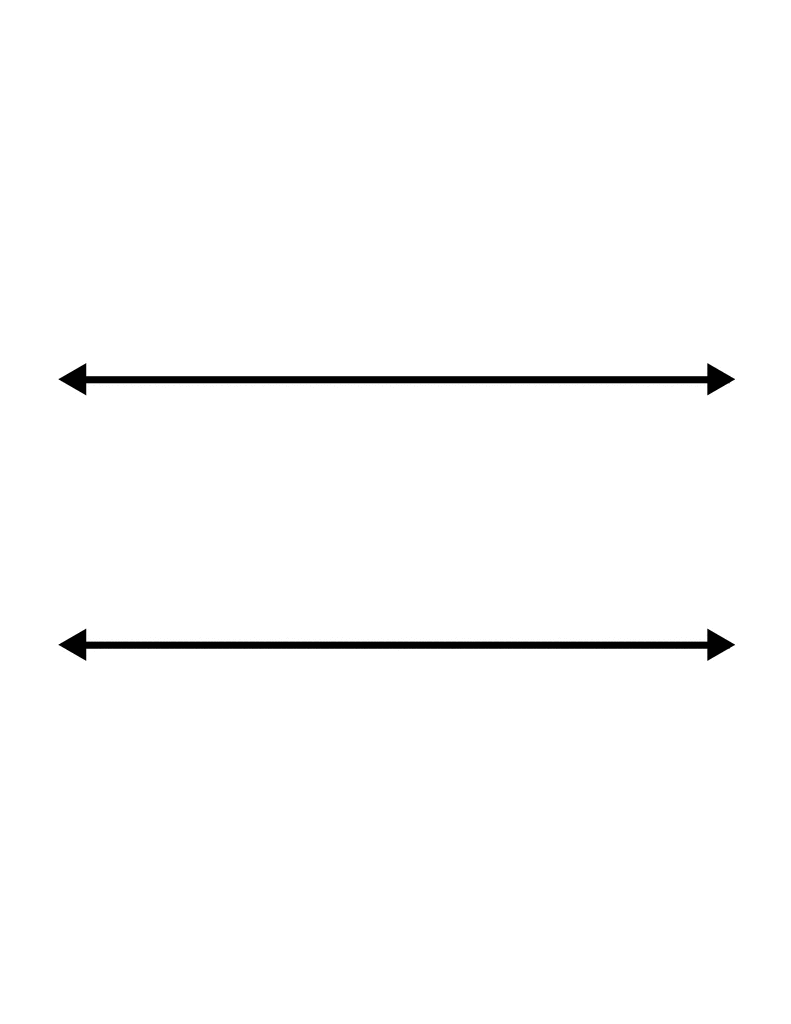Mental Math
Time Machine
Function Machines
Unit Rates
Semester 1
Trivia
50

Mental Math

100 + 900 =

1000

50

If x = 6, what is x +4

10

50

What is the output when the input is 10?20

50

What is the output when the input is 0?3

50

What is the unit rate for:

y = 10 + 1.75x ?

1.75

50

Identify this rigid transformation.A Translation

50

Gage Mascot

Gator

100

Mental Math

50 + 50 + 10

110

100

If x = 6, what is x - 7

-1

100

What is the input when the output is 27.5?7

100What is the output when the input is 1?

5

100

For a 5 oz. medium cup of frozen yogurt, Phil charges $3.00. Toppings cost$0.50 each. The unit rate is...

$0.50 100 Identify this transformation.A Rotation 100 The year the Gage Canal was completed. (hint, the answer is on the mural out front) 1889 200 5 + 5 + 5 x 5 35 200 If x = 6, what is x? 36 200 What is the start value for this graph?$45
200

What is the output when the input is 1/2?4

200

y = 4.99x  the unit rate is...

4.99

200

This has _______ solution(s).No Solution.

200

The principal of Matthew Gage Middle School

Mr. Reller

300

Mental Math

865/10

86.5

300

If x = 6, what is 1/3(x)?

2

300

According to this graph, what's the maximum number of rides you can go on?18

300

What is the output when the input is -1 ?1

300

The unit rate is...4 meters per second

300

The solution is...(2, -1)

300

The assistant principal at Matthew Gage Middle School.

Mrs. Jaggers

400

Mental Math

1048 + 202 =

1250

400

5(x + 2) ?

5x + 10

400

What is the input when the output is $35?4 rides 400 What is the output when the input is -50?-97 400 The unit rate is...$8 per car

400

The slope is...5/3

400

The year Matthew Gage Middle School was established.

1963

Click to zoom